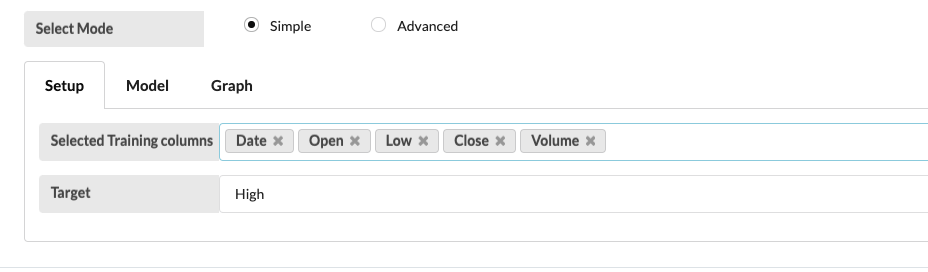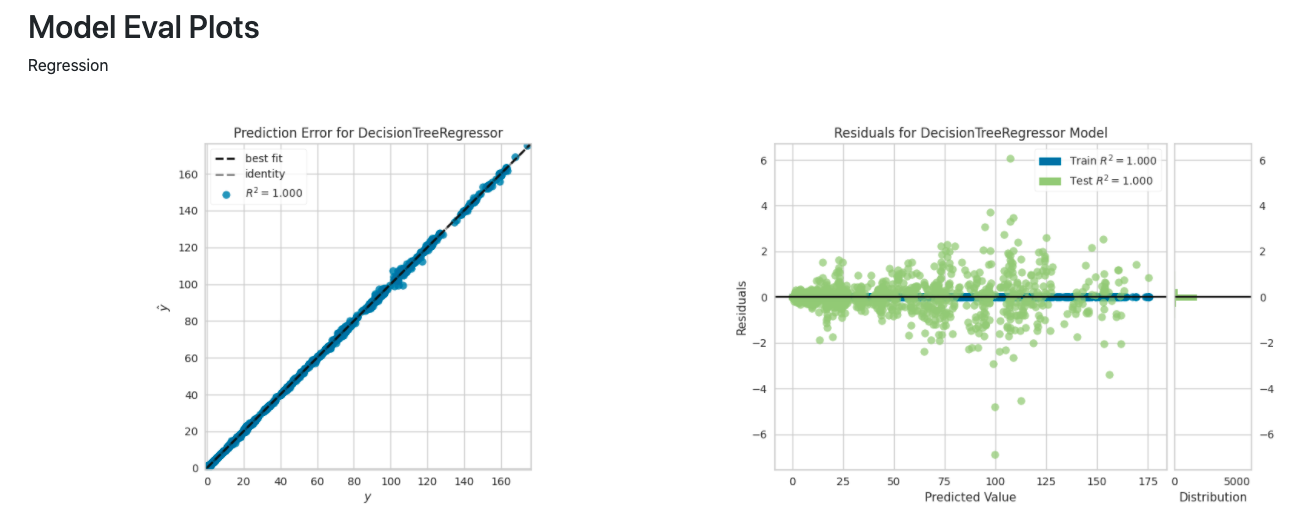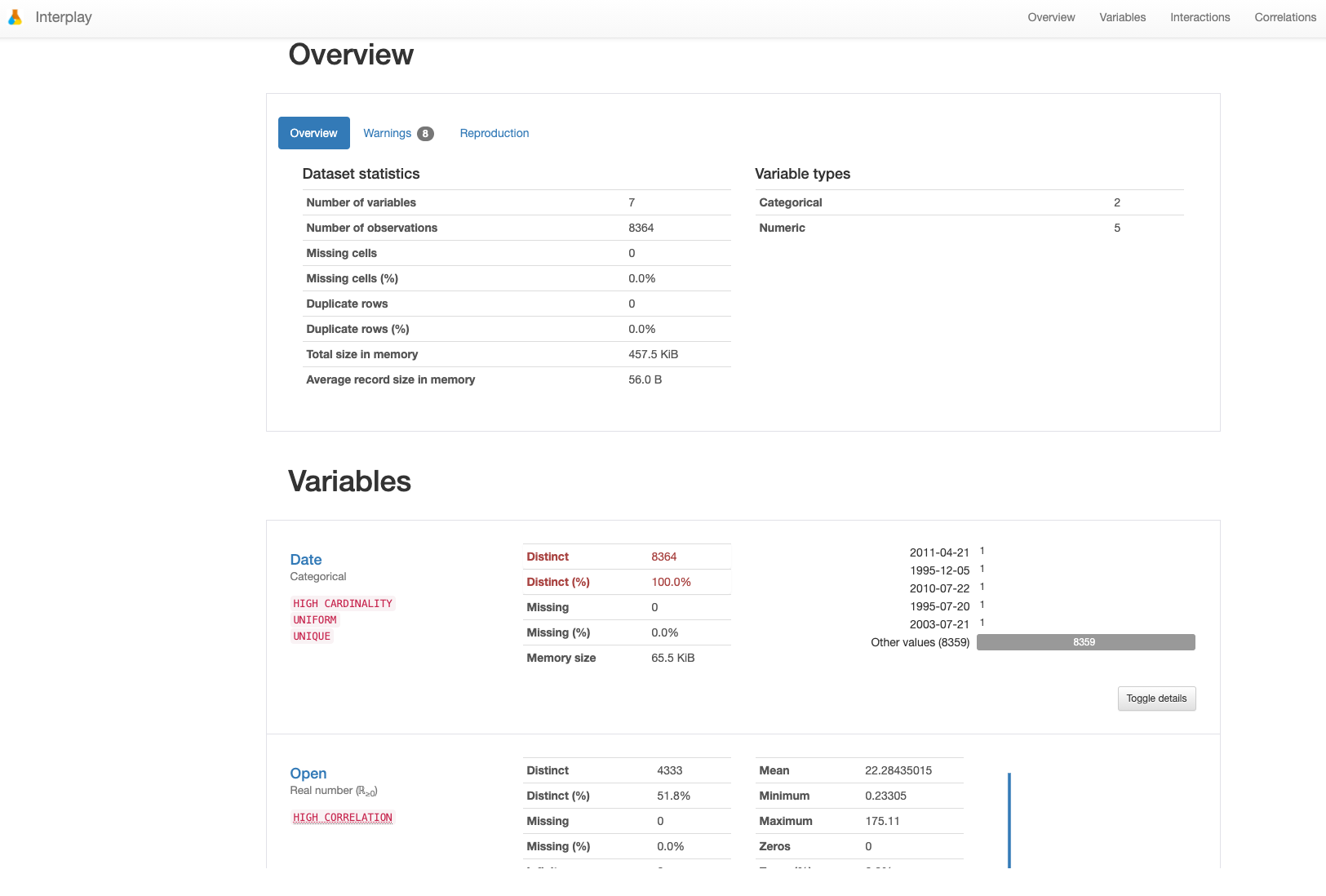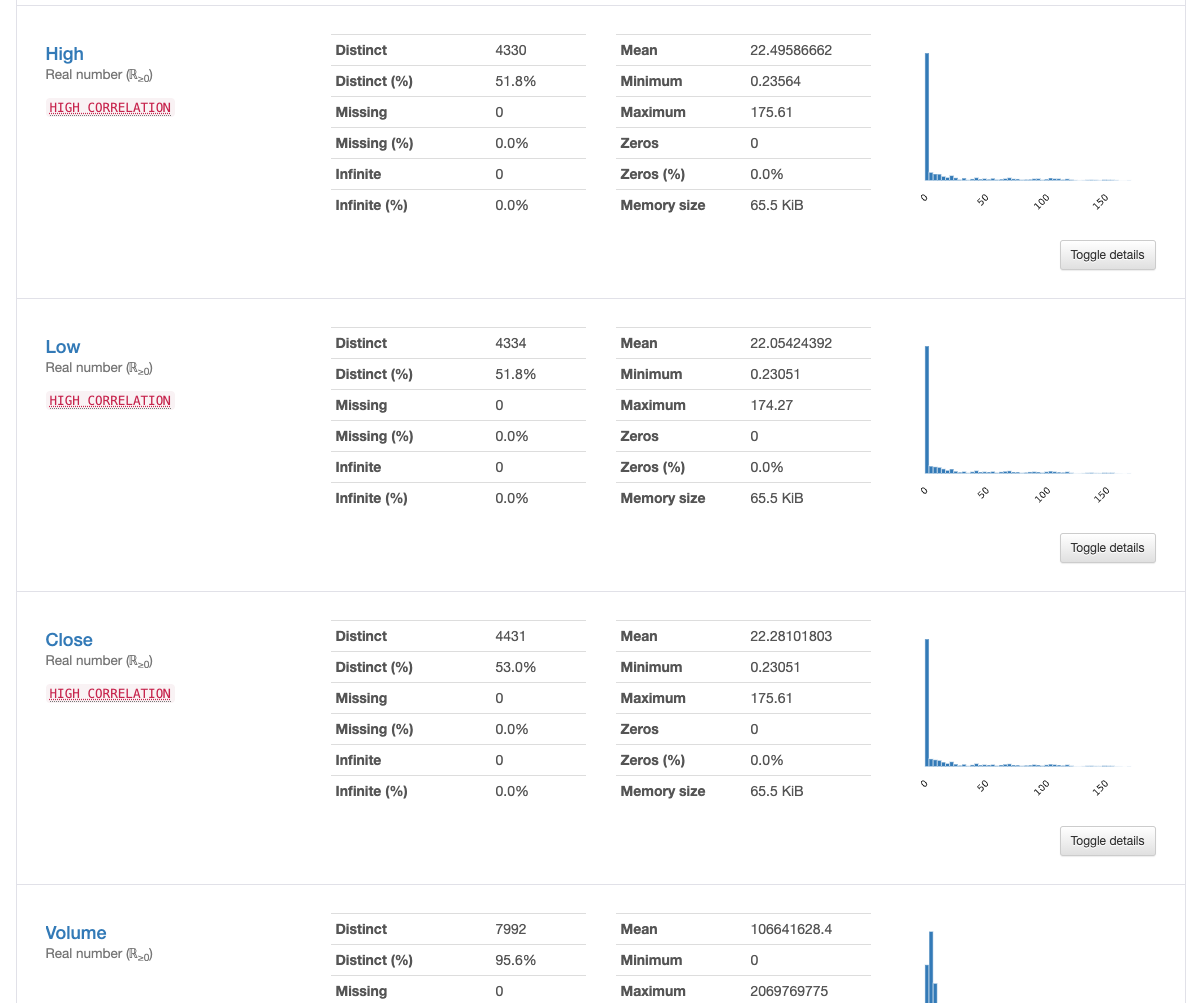Regression

Training an AI model using auto AI

Regression is a supervised machine learning technique which is used to predict continuous values. The ultimate goal of the regression algorithm is to plot a best-fit line or a curve between the data. The three main metrics that are used for evaluating the trained regression model are variance, bias and error. The main difference between regression and classification algorithms that regression algorithms are used to predict the continuous values such as price, salary, age, etc. and classification algorithms are used to predict/classify the discrete values such as Male or Female, True or False, Spam or Not Spam, etc.

In this example we will look at the we will train an AI model training and target columns such as: date, open, high, low, close, volume of Apple stock dataset from 1984 to 2017. It will be trained to predict the high over the data set it will give you a prediction and residual decision tree model. It will also give you measuring analytics such as RME, matrix, etc.

Step 1

Open an inject node and leave the payload on timestamp setting.Step 2.Open advanced regression trainer configurator node, select the apple.us.csv under standard dataset, click on get data. Select the training and target columns. Set the model to decision tree and name regression predictor Apple Stock Predict - Regression.Step 3.

Add a data visualize, model visualize and console nodes and connect it to the regression node and connect the regression node to the inject (timestamp) node and click on deploy.Step 4

Click on the timestamp/Inject node switch (grey switch) and click on the debug menu for the model to predict the data.Step 5.

Click on the model visualize switch (yellow switch) will show us the regression and residual decision tree model based it's prediction of Apple stock over the course of 33 years (1984-2017).Step 6.

Data visualize shows the overview of the variables of date, open, high, low, close of the Apple stock has performed over the course of 33 years.